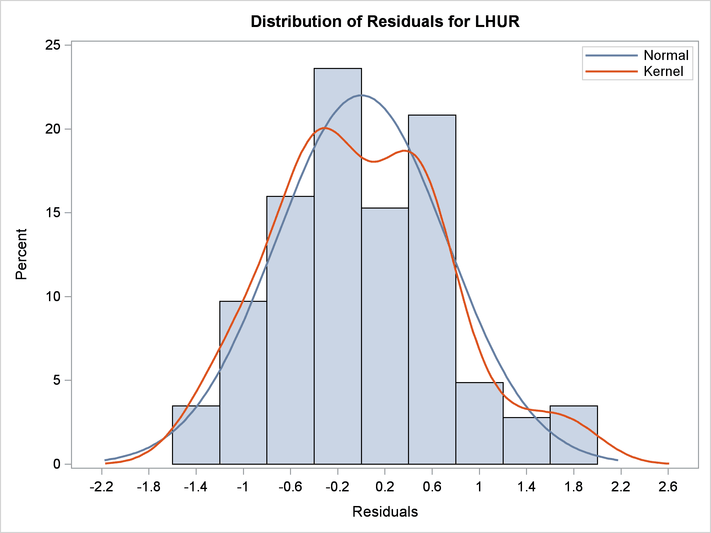# The MODEL Procedure

### Example 19.20 Illustration of ODS Graphics

This example illustrates graphical output from PROC MODEL. This is a continuation of the section Nonlinear Regression Analysis. For information about the graphics available in the MODEL procedure, see the section ODS Graphics.

The following statements show how to generate ODS Graphics plots with the MODEL procedure. The plots are displayed in Output 19.20.1 and Output 19.20.2. Note that the variable `date` in the ID statement is used to define the horizontal tick mark values when appropriate.

```title1 'Example of Graphical Output from PROC MODEL';

proc model data=sashelp.citimon;
lhur = 1/(a * ip + b) + c;
fit lhur;
id date;
run;
```

Output 19.20.1: Diagnostics Plots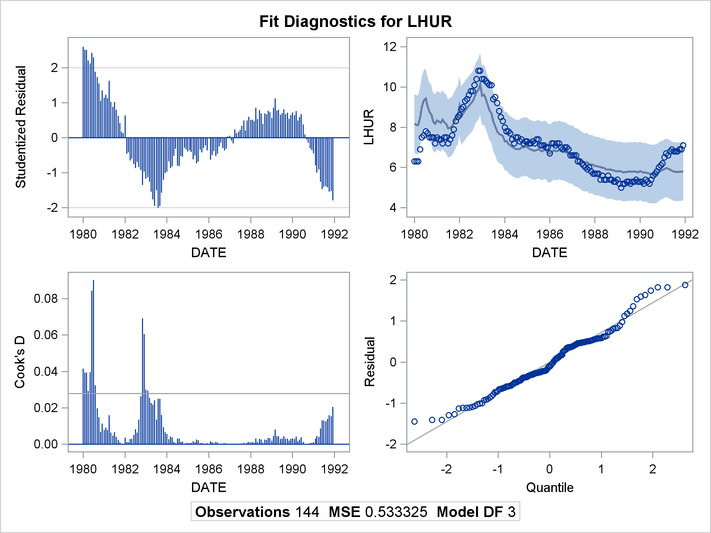Output 19.20.2: Diagnostics Plots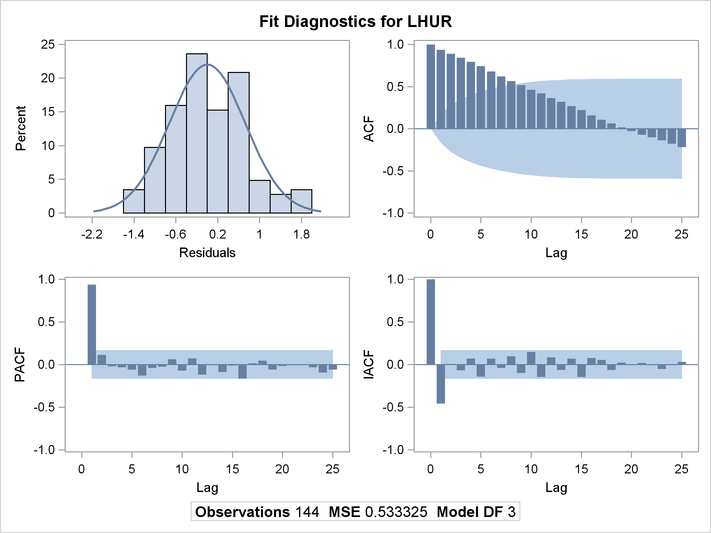You can also obtain the plots in the diagnostics panel as separate graphs by specifying the PLOTS(UNPACK) option. These plots are displayed in Output 19.20.3 through Output 19.20.10.

```title1 'Unpacked Graphical Output from PROC MODEL';

proc model data=sashelp.citimon plots(unpack);
lhur = 1/(a * ip + b) + c;
fit lhur;
id date;
run;
```

Output 19.20.3: Studentized Residuals Plot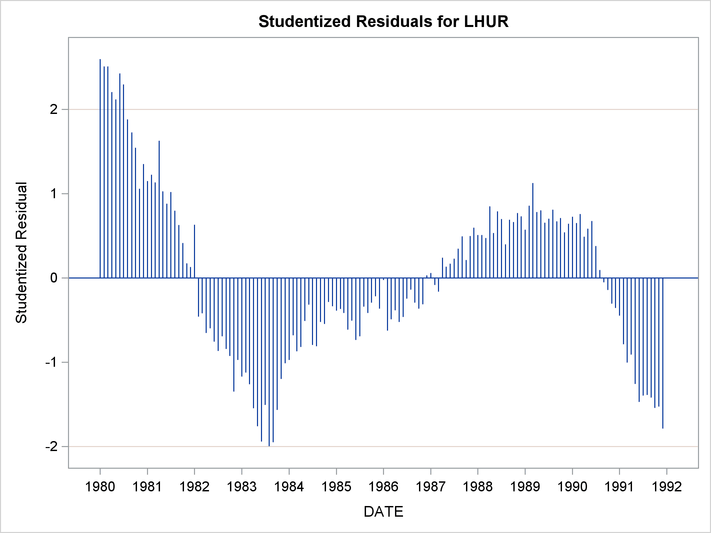Output 19.20.4: Cook’s D Plot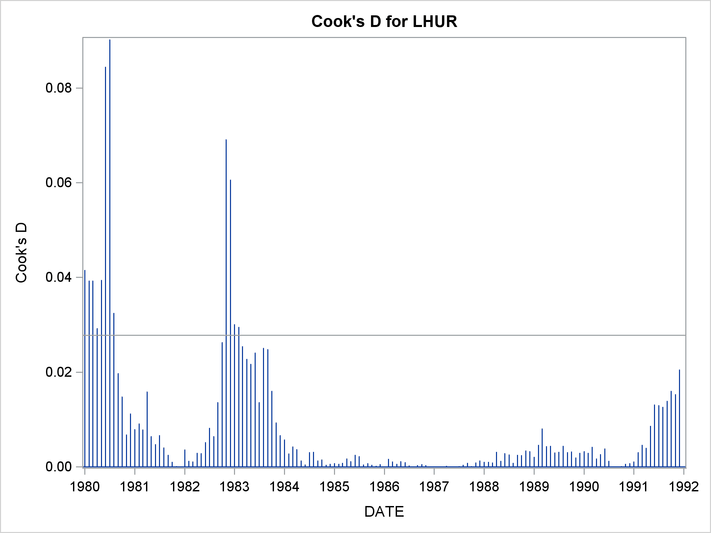Output 19.20.5: Predicted versus Actual Plot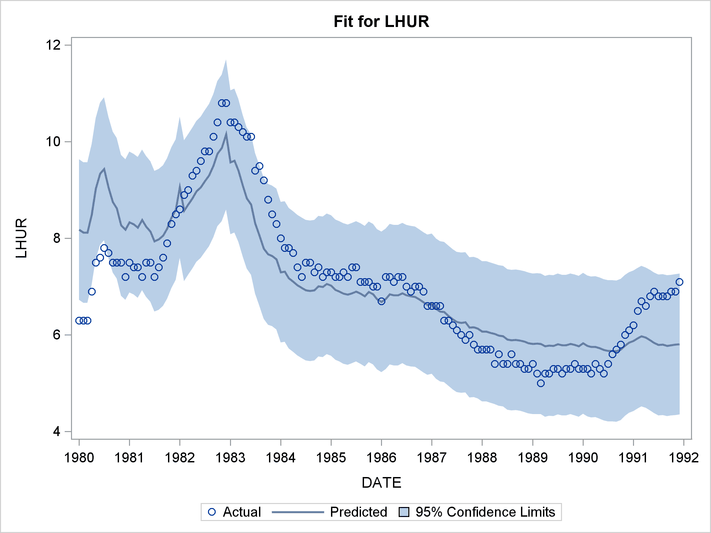Output 19.20.6: Autocorrelation of Residuals Plot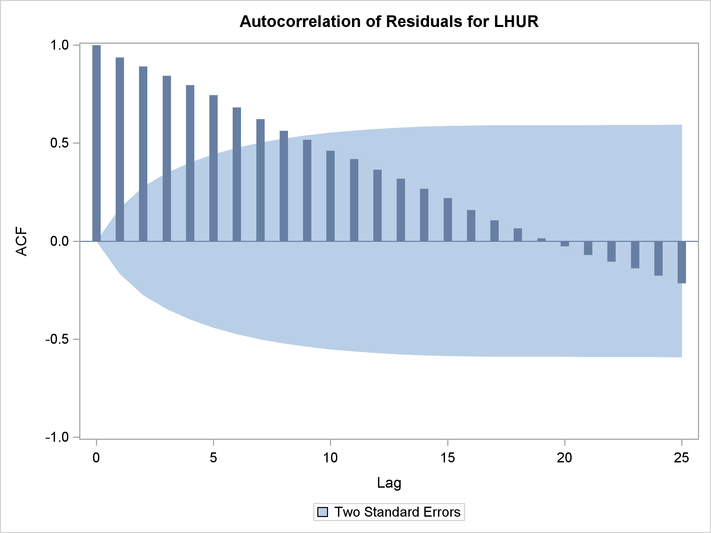Output 19.20.7: Partial Autocorrelation of Residuals Plot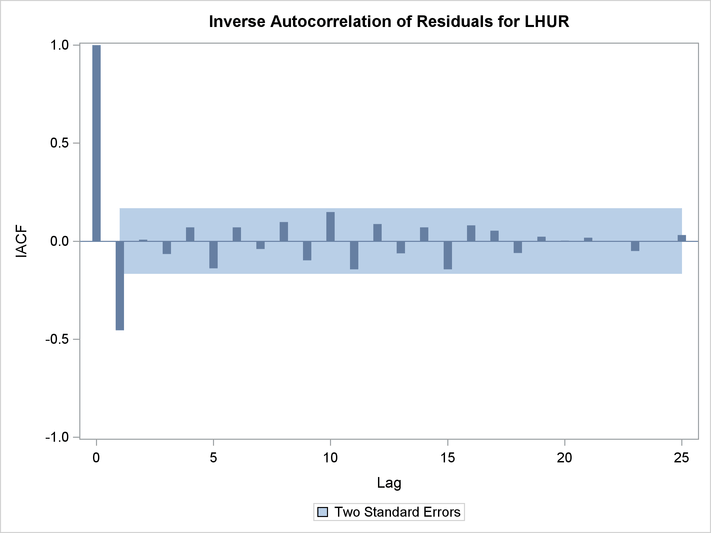Output 19.20.8: Inverse Autocorrelation of Residuals Plot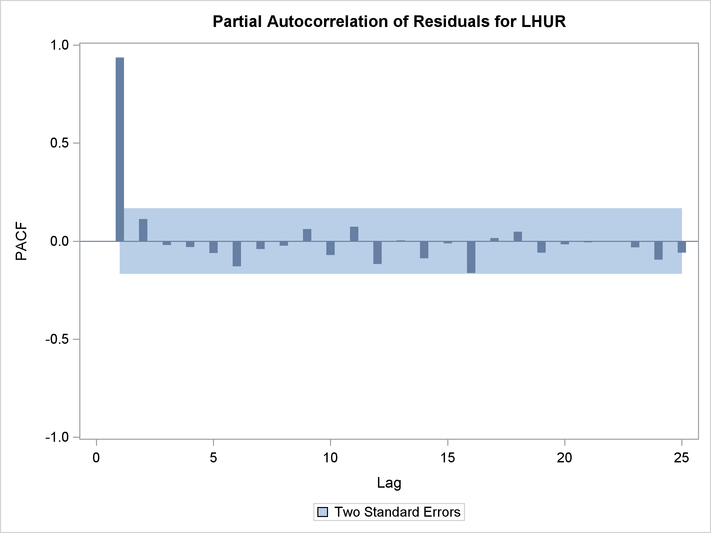Output 19.20.9: Q-Q Plot of Residuals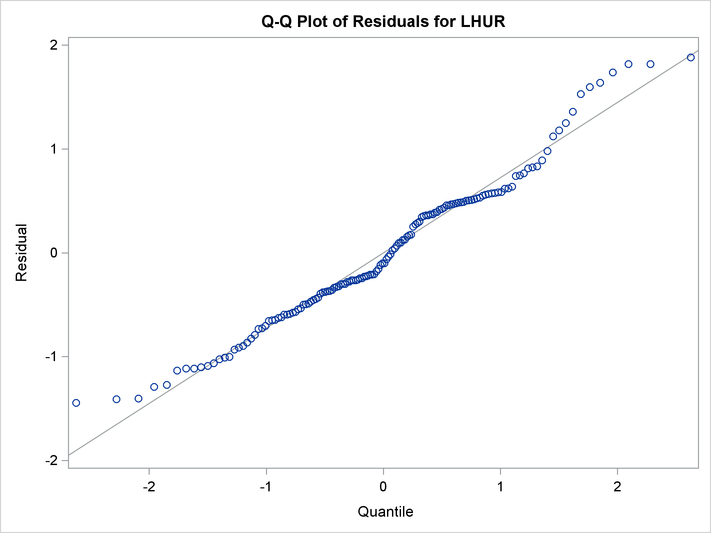Output 19.20.10: Histogram of Residuals